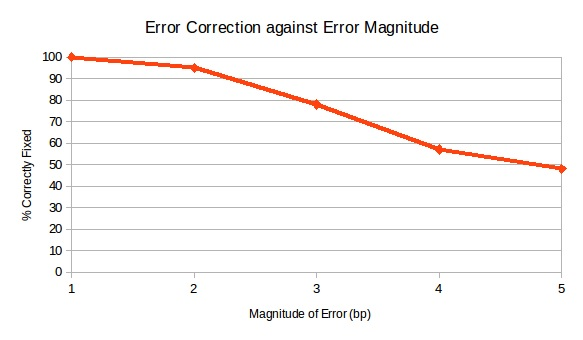# Team:Edinburgh UG/Error Correction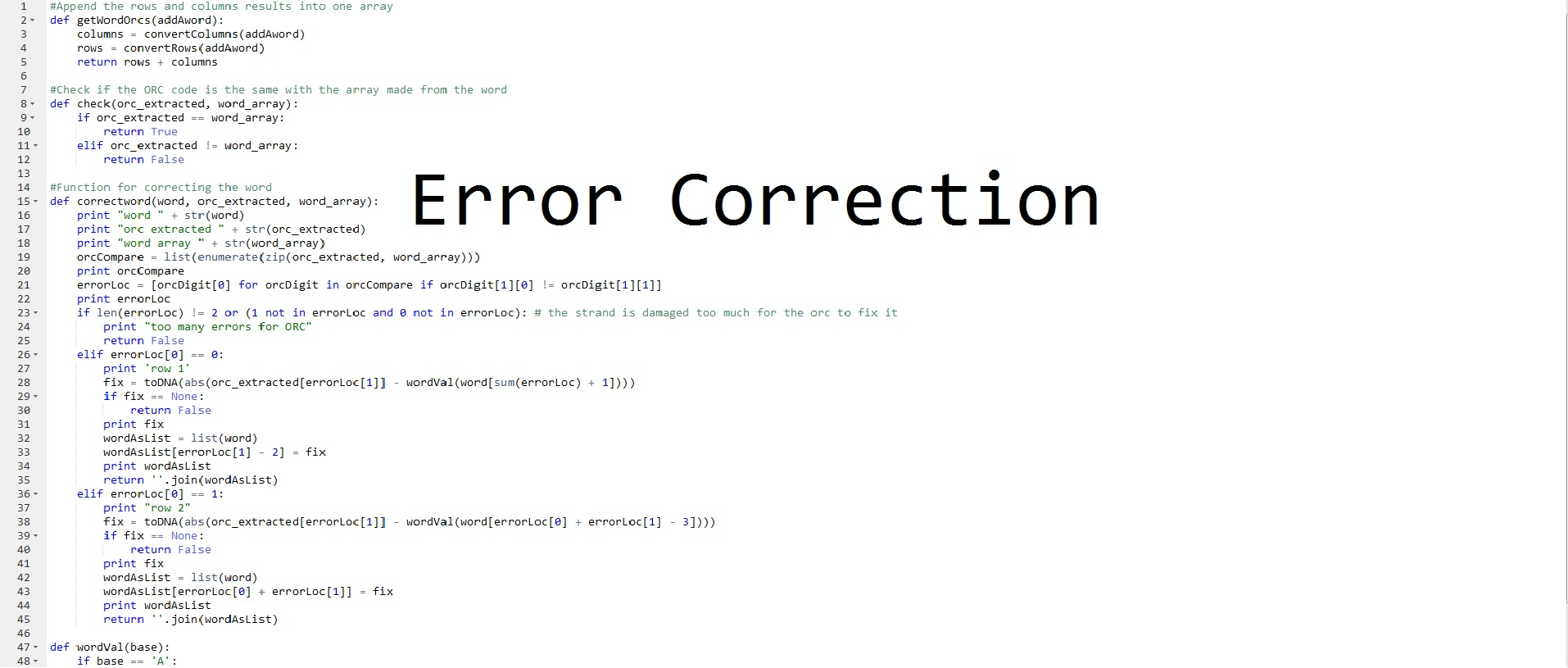In any information storage system, the primary issue is being able to read back data with as high a fidelity as possible. Storage of archival data would be futile if the medium used could not guarantee this. BabblED utilises a powerful system of error detection and correction using checksums, optimal rectangular codes and Levenshtein distance to ensure fidelity of data even when a DNA sequence is damaged or there are mistakes in sequencing. Using these and the incredible properties of DNA we can be confident in accurately storing archival data for 10s or even 100s of years. You can read more about all these mechanisms below or jump straight into the code at our Github

#### Checksum

A checksum is a commonly used technique in network engineering that we’ve adapted for use in DNA storage. In this format the checksum allows the detection of errors in a BabbleBlock (DNA sentence). The checksum holds the sum of the word coding regions of BabbleBricks (DNA words) in a BabbleBlock in a 4 BabbleBrick region that can be found towards the end of a BabbleBlock.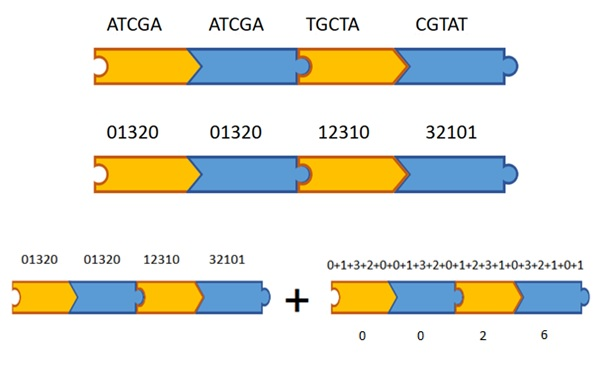When a BabbleBlock is being translated the checksum is looked at first to see if it matches the sums of the word coding regions in the DNA we get back from sequencing. If this is the case, no errors have appeared and the translation program can proceed as normal however if there is no match an error has occurred somewhere in the BabbleBlock and other error correcting mechanisms will have to be used to make sure the stored information can still be read back.

#### Improved Checksum - Collaboration with Exeter University

Fig1
Fig2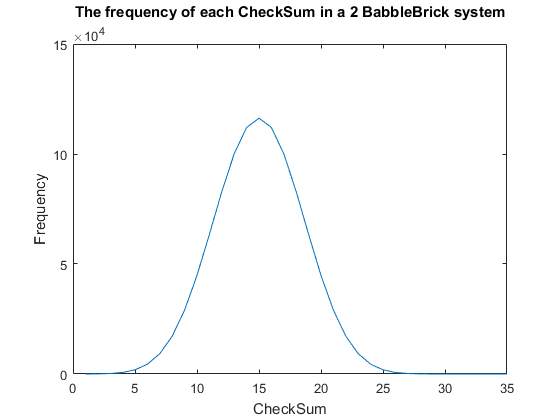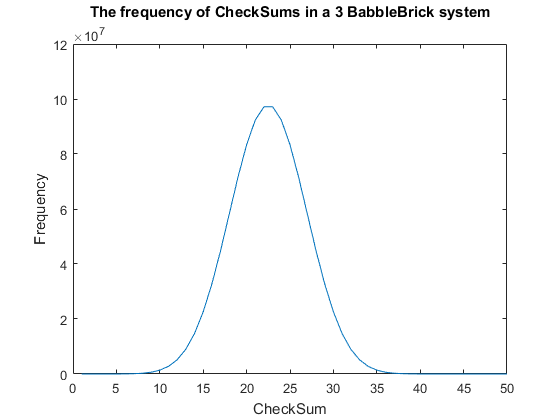Currently a checksum utilizes only a small percentage of the values that can be stored. A BabbleBrick contains 5 base 4 digits meaning that 4^5B unique bits of information share one of 15B checksums where B is the amount of BabbleBricks in one BabbleBlock. This data has been plotted for BabbleBlocks containing 2 and 3 BabbleBricks in Fig.1 and Fig.2 respectively. Assuming that between the time of writing and reading any number of mutations can occur, the maximum probability of a mutation event resulting in the same checksum can be calculated by comparing the frequency of one checksum to the total frequency of unique bits of information.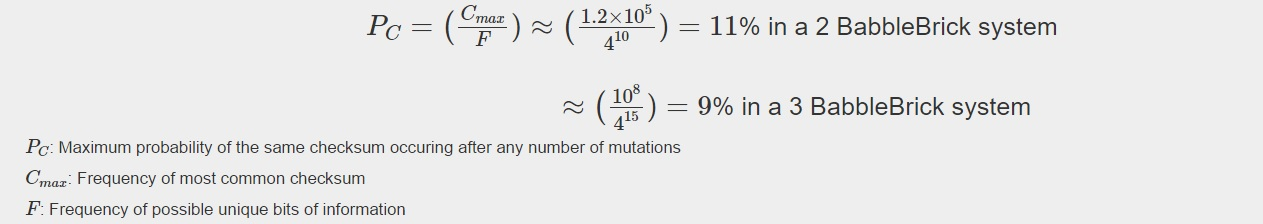Therefore, it can be predicted that for an average sentence containing 9 words the maximum probability of the same checksum occurring will be of the magnitude of 1%. The probability should decrease marginally when adding BabbleBricks due to the slightly increased range of checksums that become available. This value can be optimized by altering the method of the checksum to utilize a greater range of values and to spread out the frequency more evenly as to reduce the maximum probability of the same checksum occurring.

Currently one BabbleBlock has 4 BabbleBricks dedicated to storing the checksum, giving a maximum 10^4 possible values. The first step in determining a ‘CheckMethod’ is to ensure that all checksums for a suitable amount of BabbleBricks can be stored without going over 10^4. It is also important to not use operators that will result in negative numbers or decimals, therefore limiting the possible checksum values to integers up to but not including 10^4, this rules out operators such as subtract and divide. For this example, a suitable number of words in a sentence and therefore BabbleBricks in a BabbleBlock shall be 20. All simulations will be carried out on 3 BabbleBrick systems due to computing limitations.

Checksums are non-directional, for example a BabbleBrick of bases [2,2,2,2,2] would have the same checksum as [2,1,3,2,2]. To alter this a checkmethod will incorporate the position of the base in to the calculation. At each point the digit is multiplied by its position in the BabbleBlock, where the first BabbleBrick has digit positions 1 to 5 and the last BabbleBrick (20th}) has positions 96 to 100. A scaler alpha has been included to increase the range of results. To ensure multiplications don’t result in a null result the value of each base had a value of 1 added to it. The first checkmethod of one BabbleBlock can be defined as: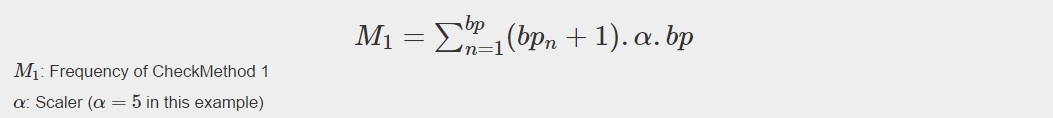Fig3
Fig4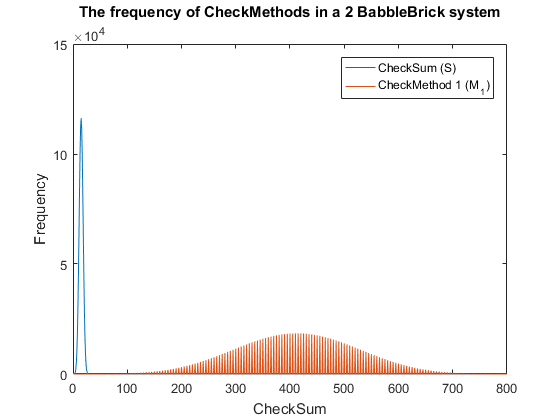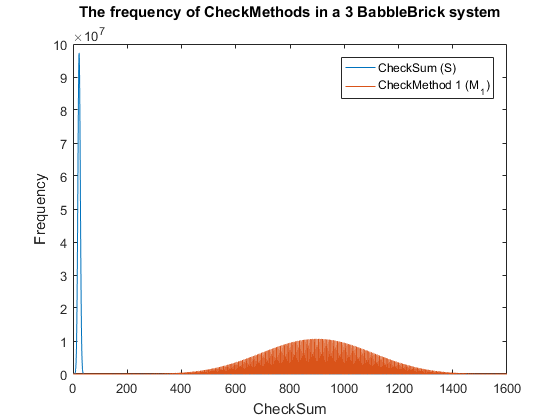This method results in Fig.3 and Fig.4 for a 2 and 3 BabbleBlock system respectively, which shows a large improvement over the original checksum method. The maximum frequency of a single checksum has been significantly decreased which will lower the probability of a false positive occurring; this is largely due to the large range of results available to the method. However, there is still room for improvement as the shaded area of the graph indicates that on a smaller scale the frequency of checkmethod 1 varies between high and low values. Eliminating this fluctuation would allow for the data to be spread out more evenly. To improve this method a second layer of multiplication will be implemented, each digit will now be multiplied by a constant depending on its relative position in the BabbleBrick.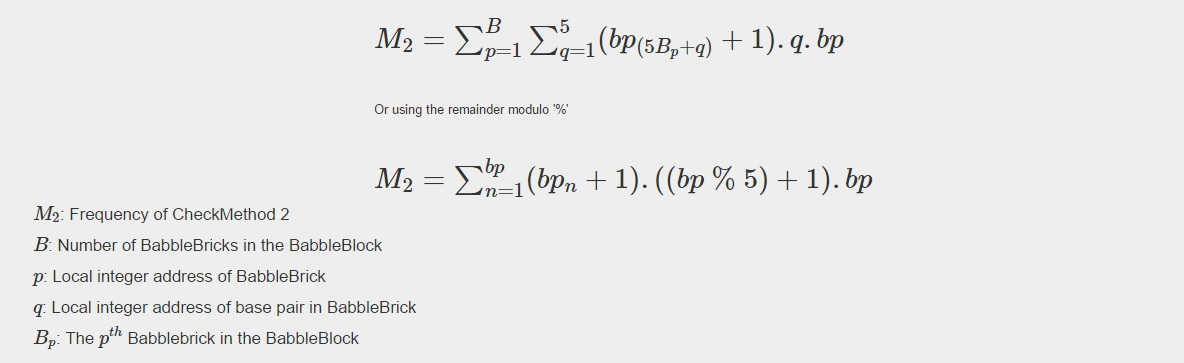Fig5
Fig6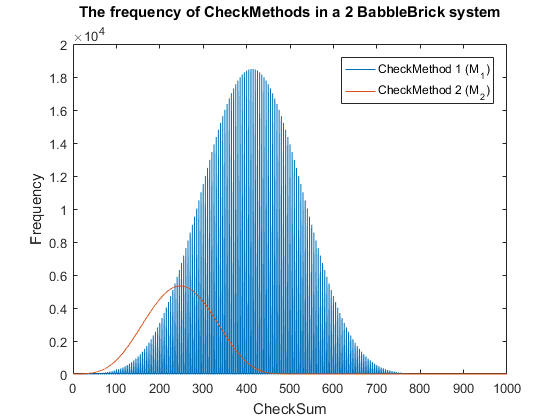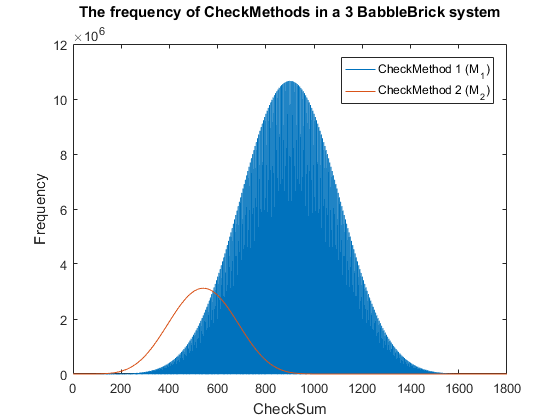This has been plotted for a 2 and 3 BabbleBlock system in Fig.5 and Fig.6 respectively. When comparing checksum to checkmethod 2 the frequency peak is approximately 20 to 30 times smaller in both cases whilst utilizing more values. In Fig.5 and Fig.6 the largest improvement using the second iteration of the checkmethod is the utilization of every integer value, checkmethod 1 appears shaded as the frequency varies frequently. The last step is to test checkmethod 2 when used in a babbleBlock containing 20 BabbleBricks; the largest value possible assuming a BabbleBlock containing the value ‘3’ in each digit will grant a value of 60600 which falls out of the current limit of 10^4 values. Therefore, it is recommended that one more BabbleBrick is added to the end of the BabbleBlock in order to store 10^5 values.

To improve this method further more complex multiplications could be added, it would be a decision based on optimising efficiency of calculations and minimising false positives. In a 2 and 3 BabbleBrick system the probability of a false positives occurring was reduced by approximately 20 and 30 times respectively, although the numbers are too large to compute, this new method has the possibility of lowering the maximum false positive error of the previously used checksum by one or more orders of magnitude.

#### Optimal Rectangular Code

The Optimal Rectangular Code (ORC) is a type of error correcting code that is present in all of our BabbleBricks. When the checksum detects an error the next action is to checks the ORCs in each word and use them to correct any errors that have taken place as follows: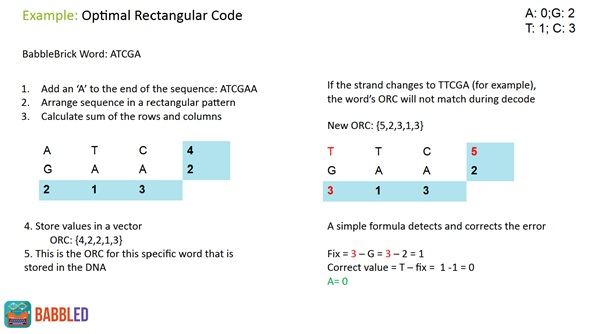Unfortunately, the ORC can only locate and fix single base pair errors however the properties of the ORC facilitate another added level of error correction.

#### Beyond Optimal Rectangular Codes - The SuperFixer Function and Levenshtein Distance

If the Optimal Rectangular Code (ORC) detects more than the single base pair error it can fix using its normal actions the SuperFixer function is called. The SuperFixer uses the ORC and works out all the possible words in the lexicon that fit this pattern. For any given ORC this is a maximum of 4 words and indeed there are many words that have unique ORC’s. Once the SuperFixer has narrowed down the possible words the list of possibilities is compared to what appears in the actual DNA and the sequence with the lowest Levenshtein distance is used in the translation. Levenshtein distance is a metric which shows the number of deletions, insertions or substitutions it will take to turn one string (or sequence) into another.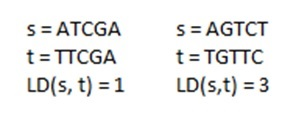By taking the minimum Levenshtein distance we always work under the assumption that the smallest number of errors is most probable. This assumption gives the best performance across errors of different magnitudes due to the robustness of DNA as a molecule making small errors considerably more likely than larger ones.

#### Overall Performance

Finally, this graph represents the performance of our entire error correction system on errors of varying magnitudes: